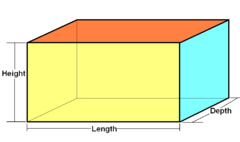# Cuboid

﻿
Cuboid

In geometry, a cuboid is a solid figure bounded by six faces, forming a convex polyhedron. There are two competing (but incompatible) definitions of a cuboid in mathematical literature. In the more general definition of a cuboid, the only additional requirement is that these six faces each be a quadrilateral, and that the undirected graph formed by the vertices and edges of the polyhedron should be isomorphic to the graph of a cube. Alternatively, the word “cuboid” is sometimes used to refer to a shape of this type in which each of the faces is a rectangle (and so each pair of adjacent faces meets in a right angle); this more restrictive type of cuboid is also known as a right cuboid, rectangular box, rectangular hexahedron, right rectangular prism, or rectangular parallelepiped.

## General cuboids

By Euler's formula the number of faces ('F'), vertices (V), and edges (E) of any convex polyhedron are related by the formula "F + V - E" = 2 . In the case of a cuboid this gives 6 + 8 - 12 = 2; that is, like a cube, a cuboid has 6 faces, 8 vertices, and 12 edges.

Along with the rectangular cuboids, any parallelepiped is a cuboid of this type, as is a square frustum (the shape formed by truncation of the apex of a square pyramid).

## Rectangular cuboid

Rectangular CuboidType Prism
Faces 6 rectangles
Edges 12
Vertices 8
Symmetry group D2h, [2,2], (*222)
Schläfli symbol {}x{}x{}
Coxeter-Dynkin diagramProperties convex, zonohedron, isogonal

In a rectangular cuboid, all angles are right angles, and opposite faces of a cuboid are equal. It is also a right rectangular prism. The term "rectangular or oblong prism" is ambiguous. Also the term rectangular parallelepiped or orthogonal parallelepiped is used.

The square cuboid, square box, or right square prism (also ambiguously called square prism) is a special case of the cuboid in which at least two faces are squares. The cube is a special case of the square cuboid in which all six faces are squares.

If the dimensions of a cuboid are a, b and c, then its volume is abc and its surface area is 2ab + 2bc + 2ac.

The length of the space diagonal is$d = \sqrt{a^2+b^2+c^2}.\$

Cuboid shapes are often used for boxes, cupboards, rooms, buildings, etc. Cuboids are among those solids that can tessellate 3-dimensional space. The shape is fairly versatile in being able to contain multiple smaller cuboids, e.g. sugar cubes in a box, small boxes in a large box, a cupboard in a room, and rooms in a building.

A cuboid with integer edges as well as integer face diagonals is called an Euler brick, for example with sides 44, 117 and 240. A perfect cuboid is an Euler brick whose space diagonal is also an integer. It is currently unknown whether a perfect cuboid actually exists.

Wikimedia Foundation. 2010.

### Look at other dictionaries:

• Cuboid — Éditeur Creat Studios et TikGames Développeur Creat Studios Date de sortie …   Wikipédia en Français

• cuboid — cuboíd s. n., adj. m., pl. cuboízi; f. sg. cuboídă, pl. cuboíde Trimis de siveco, 10.08.2004. Sursa: Dicţionar ortografic ﻿ CUBOÍD, Ă adj. De formă aproximativ cubică; (şi …   Dicționar Român

• Cuboid — Cu boid (k? boid), a. [Cube + oid: cf. Gr. ???.] (Anat.) Cube shaped, or nearly so; as, the cuboid bone of the foot. n. (Anat.) The bone of the tarsus, which, in man and most mammals, supports the metatarsals of the fourth and fifth toes. [1913… …   The Collaborative International Dictionary of English

• cuboid — [kyo͞o′boid΄] adj. [Gr kyboeidēs: see CUBE1 & OID] 1. shaped like a cube 2. designating a cubelike tarsal bone on the outside of the foot between the metatarsals and the calcaneus n. 1. a six sided figure each face of which is a rectangle …   English World dictionary

• cuboid — (adj.) cube like, 1829, a modern coinage; see CUBE (Cf. cube) (n.) + OID (Cf. oid) …   Etymology dictionary

• cuboid — ► ADJECTIVE ▪ more or less cubic in shape. ► NOUN ▪ a solid which has six rectangular faces at right angles to each other. DERIVATIVES cuboidal adjective …   English terms dictionary

• cuboid — cu·boid kyü .bȯid adj 1) relating to or being the cuboid <the cuboid bone> 2) shaped approximately like a cube cuboid n the outermost bone in the distal row of tarsal bones of the foot that supports the fourth and fifth metatarsals * * *… …   Medical dictionary

• cuboid — I UK [ˈkjuːbɔɪd] / US [ˈkjubɔɪd] noun [countable] Word forms cuboid : singular cuboid plural cuboids maths a shape like a box that is similar to a cube, but with surfaces that are rectangles, not squares II UK [ˈkjuːbɔɪd] / US [ˈkjubɔɪd]… …   English dictionary

• cuboid — I. adjective Date: circa 1828 1. approximately cubical in shape 2. relating to or being the cuboid II. noun Date: 1839 the outermost bone in the distal row of tarsal bones of many higher vertebrates …   New Collegiate Dictionary

• cuboid — /kyooh boyd/, adj. 1. Also, cuboidal. 2. resembling a cube in form. 3. Anat. noting or pertaining to the outermost bone of the distal row of tarsal bones. n. 4. Math. a rectangular parallelepiped. 5. Anat. the cuboid bone. [1700 10; < Gk… …   Universalium